Performance tunning is becoming a key focus for most of the DBAs. In scope of the “dbi services labs”, I made some tests on functions which can help improving these, but which are not always well-known. Today’s article is going to describe one of these, the SPARE COLUMNS.
The property of Spare columns is not the most used in client environment that we have seen.
This article present a script to find the potential columns that can have the sparse property with the usage restrictions to make a space tuning in your database and especially for Data Warehouse (DWH).

The database example that we used, is AdventureWorksDW2008R2.

### Principle

The msdn explain very well the principle. I will just resume it:
“Sparse columns reduce the space requirements for null values at the cost of more overhead to retrieve non null values”

### Step 1: Create a temporary table

A temporary table is created to retrieve information about the columns of the tables in the database being audited. This table provides the following information:

• Name of th column
• Column ID
• Table name
• Table ID
• Type of the column
• If column is a sparse column
• If column is nullable
• Number of row in this table
• Number of null row in the column
• Ratio Nb NULL Rows / Nb Rows
```CREATE TABLE #temp (
ColumnName varchar(50),
ColumnID int,
TableName varchar(50),
TableId int,
TypeName varchar(50),
IsParse bit,
IsNullable bit,
NumberOfRow int,
NumberOfRowNULL int,
Ratio int)
SET NOCOUNT ON```

Note that “SET NOCOUNT ON” option is used. In the Best Practice, setting SET NOCOUNT to ON can provide a significant performance boost, as network traffic is greatly reduced.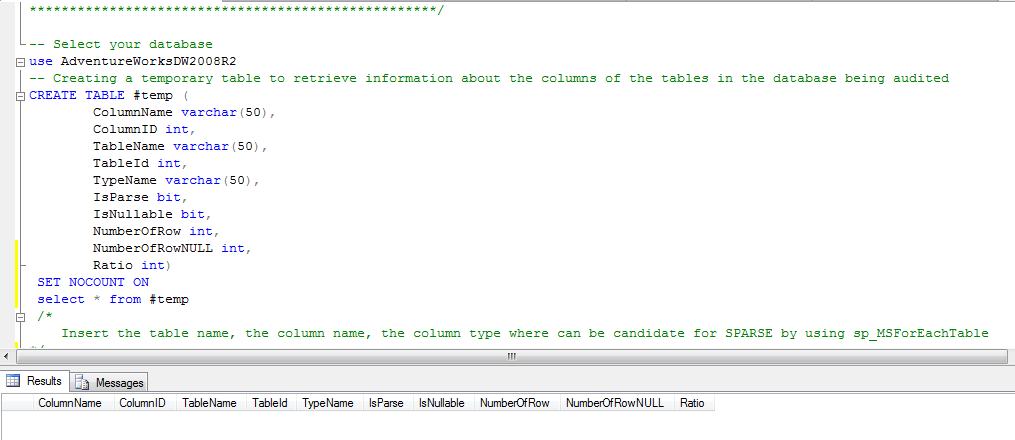### Step 2: Insert the first information and introduction to some usage’s restrictions

We insert in the temporary table the table name, the column name and the column type which can be candidate for SPARSE by using sp_MSForEachTable.

```INSERT #temp
EXEC sp_MSForEachTable '
SELECT DISTINCT
sys.columns.name ColumnName,
sys.columns.column_id ColumnID,
SUBSTRING(''?'', 8, Len(''?'')-8) AS TableName,
sys.columns.object_id TableID,
CASE systypes.name
WHEN ''sysname'' THEN ''nvarchar''
ELSE systypes.name
END AS TypeName,
sys.columns.is_sparse IsParse,
sys.columns.is_nullable IsNullable,
0,0,0
FROM sys.columns (NoLock)
INNER JOIN systypes (NoLock) ON systypes.xtype = sys.columns.system_type_id
WHERE sys.columns.object_id = (
SELECT id FROM sysobjects (NoLock)
WHERE name = SUBSTRING(''?'', 8, Len(''?'')-8))'```

But, we can optimize this query with a first part of the usage restrictions for the properties of Spare columns:

• The following data types cannot be specified as SPARSE: geography, geometry, image, ntext, text and timestamp

systypes.name NOT IN (”geography”, ”geometry”, ”image”, ”ntext”, ”text”, ”timestamp”)

• A sparse column must be nullable and cannot have the ROWGUIDCOL or IDENTITY properties.

sys.columns.is_nullable = 1
sys.columns.is_rowguidcol = 0
sys.columns.is_identity = 0

• A sparse column cannot have the FILESTREAM attribute

sys.columns.is_filestream = 0

• A sparse column cannot have a default value

sys.columns.default_object_id = 0

• A sparse column cannot be bound to a rule

sys.columns.rule_object_id = 0

• Although a computed column can contain a sparse column, a computed column cannot be marked as SPARSE

sys.columns.is_computed = 0

• A sparse column cannot be part of a user-defined table type

sys.columns.system_type_id=sys.columns.user_type_id

• And if the columns have the Spare properties, naturally we don’t count this columns

sys.columns.is_sparse = 0

The new query with the restricted usage is :

```INSERT #temp
EXEC sp_MSForEachTable '
SELECT DISTINCT
sys.columns.name ColumnName,
sys.columns.column_id ColumnID,
SUBSTRING(''?'', 8, Len(''?'')-8) AS TableName,
sys.columns.object_id TableID,
CASE systypes.name
WHEN ''sysname'' THEN ''nvarchar''
ELSE systypes.name
END AS TypeName,
sys.columns.is_sparse IsParse,
sys.columns.is_nullable IsNullable,
0,0,0
FROM sys.columns (NoLock)
INNER JOIN systypes (NoLock) ON systypes.xtype = sys.columns.system_type_id
WHERE sys.columns.object_id = (
SELECT id FROM sysobjects (NoLock)
WHERE name = SUBSTRING(''?'', 8, Len(''?'')-8)
and systypes.name NOT IN (''geography'', ''geometry'', ''image'', ''ntext'', ''text'', ''timestamp'')
and sys.columns.is_sparse = 0
and sys.columns.is_nullable = 1
and sys.columns.is_rowguidcol = 0
and sys.columns.is_identity = 0
and sys.columns.is_computed = 0
and sys.columns.is_filestream = 0
and sys.columns.default_object_id = 0
and sys.columns.rule_object_id = 0
and sys.columns.system_type_id=sys.columns.user_type_id
)'```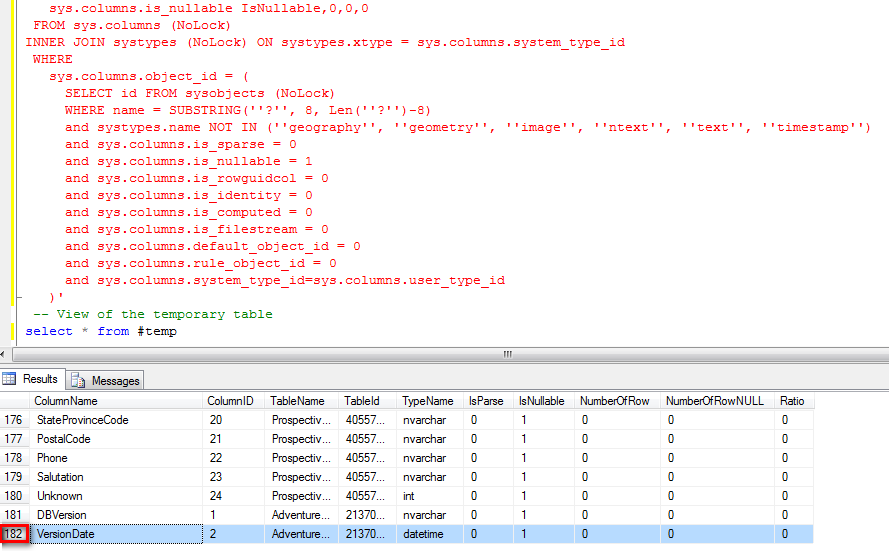In our example, we have 182 rows for the databases AdventureWorksDW2008R2

### Step 3: Usage restrictions on Index, PK and data compression

This query deletes all columns that can be a part of a clustered index or a unique primary key(PK) index :

```delete tps from #temp tps where exists (
select DISTINCT 'Exists' from sys.columns
inner join sys.indexes i on i.object_id = tps.TableId
inner join sys.index_columns ic on ic.column_id = tps.ColumnID inner join sys.columns c on c.object_id = tps.TableId and ic.column_id = c.column_id
where i.type =1 or i.is_primary_key = 1)```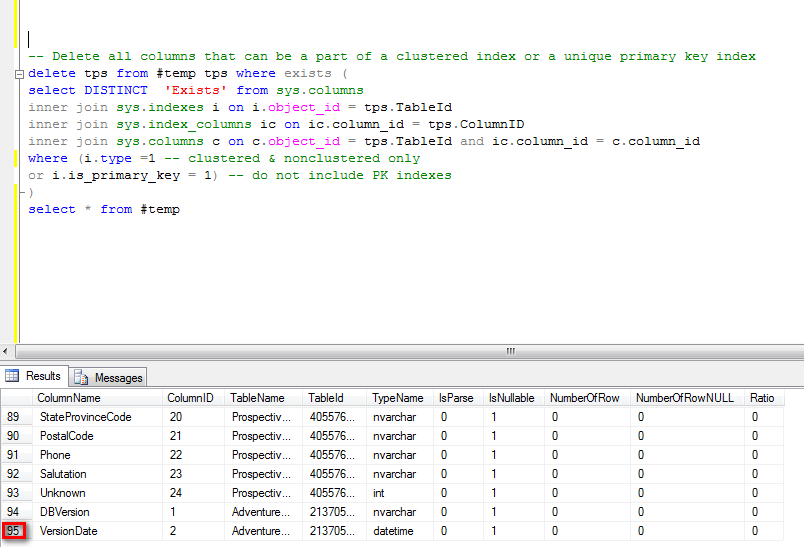We reduce the number of row at 95 in this sample.
Sparse columns are incompatible with data compression
To see if data compression is active, you can use this query:

```SELECT partition_id,
OBJECT_NAME(object_id) AS objectname,
data_compression_desc,data_compression
FROM sys.partitions where data_compression<>0;```We can see that one table (DimEmployee) use ROW Compression.
To delete columns with data compression, we use this query:

```delete tps from #temp tps inner join sys.partitions p
on p.object_id = tps.TableId where p.data_compression<>0;```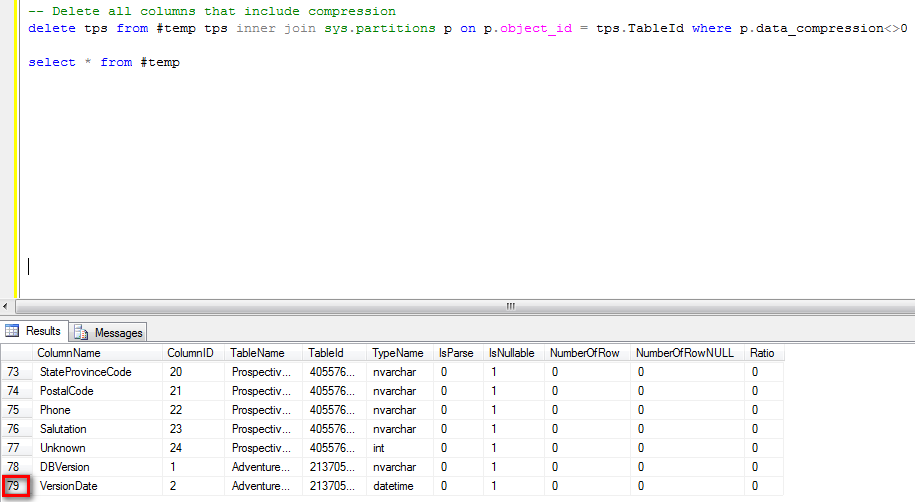We reduce the number of row at 79.

### Step 4: Add count information for the selected columns

In this step, we will get the information about the totally number of row, number of row with a “null” value and calculate the ratio in % between these 2 values.

```DECLARE @TableName nvarchar(1000)
DECLARE @ColumnName nvarchar(1000)
DECLARE @vQuery nvarchar(1000)
DECLARE @result1 INT
DECLARE @result2 INT
DECLARE tables_cursor CURSOR FAST_FORWARD
FOR
SELECT TableName,ColumnName FROM #temp
OPEN tables_cursor
FETCH NEXT FROM tables_cursor INTO @TableName,@ColumnName
WHILE @@FETCH_STATUS = 0
BEGIN
-- Search the number of row in a table
SET @vQuery = 'SELECT @result1= COUNT(*) FROM [' + @TableName + ']'
EXEC SP_EXECUTESQL
@Query = @vQuery
, @Params = N'@result1 INT OUTPUT'
, @result1 = @result1 OUTPUT
-- Search the number of row in a table
SET @vQuery = 'SELECT @result2= COUNT(*) FROM [' + @TableName + '] where [' + @ColumnName + '] is null'
EXEC SP_EXECUTESQL
@Query = @vQuery
, @Params = N'@result2 INT OUTPUT'
, @result2 = @result2 OUTPUT
update #temp set NumberOfRow = @result1,NumberOfRowNULL = @result2,Ratio = (@result2*100/@result1) where
[email protected] and [email protected]
FETCH NEXT FROM tables_cursor INTO @TableName,@ColumnName
END
CLOSE tables_cursor
DEALLOCATE tables_cursor```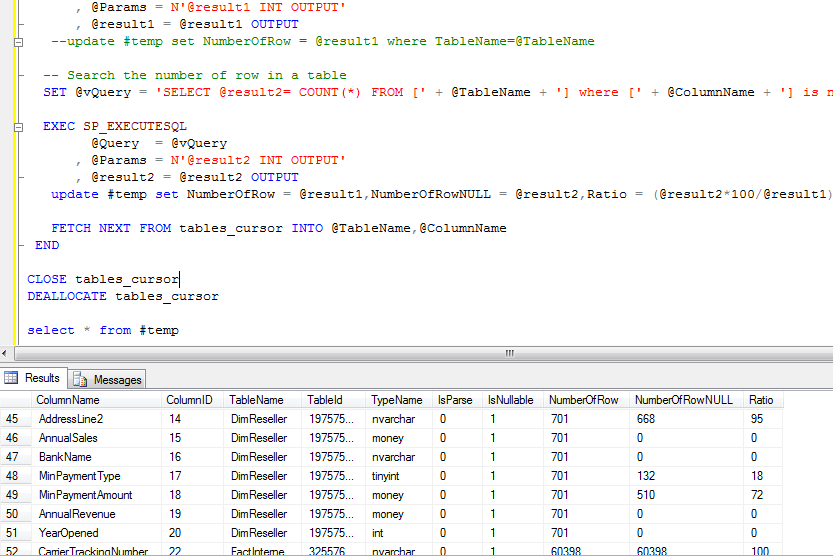For the end, we delete all line that not has a NULL value in NumberOfRowNULL and NumberOfRow columns :

`delete tps from #temp tps where NumberOfRowNULL = 0 or NumberOfRow = 0`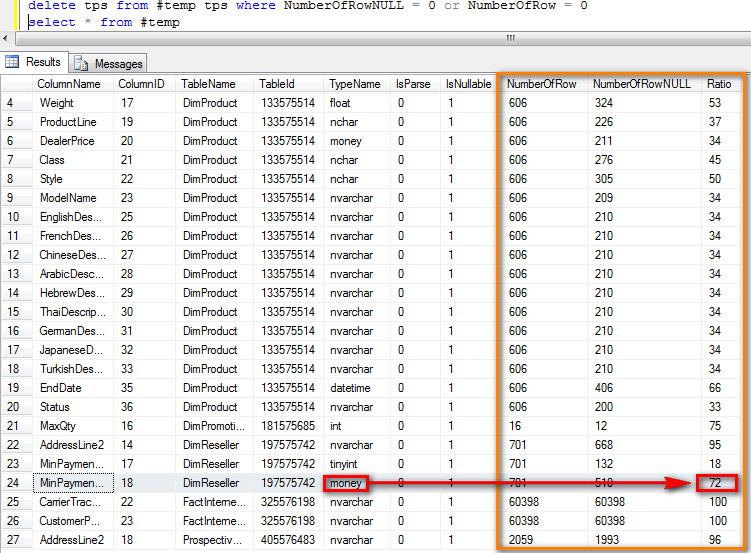### Conclusion

In this example, you can see that the column MinPaymentAmount from the table DimReseller has 72% of Null value.
In the msdn web site, you can see that for a money type, we win 40% for 52% of null value.

This is clearly a good candidate to be SPARE column.

msdn extract :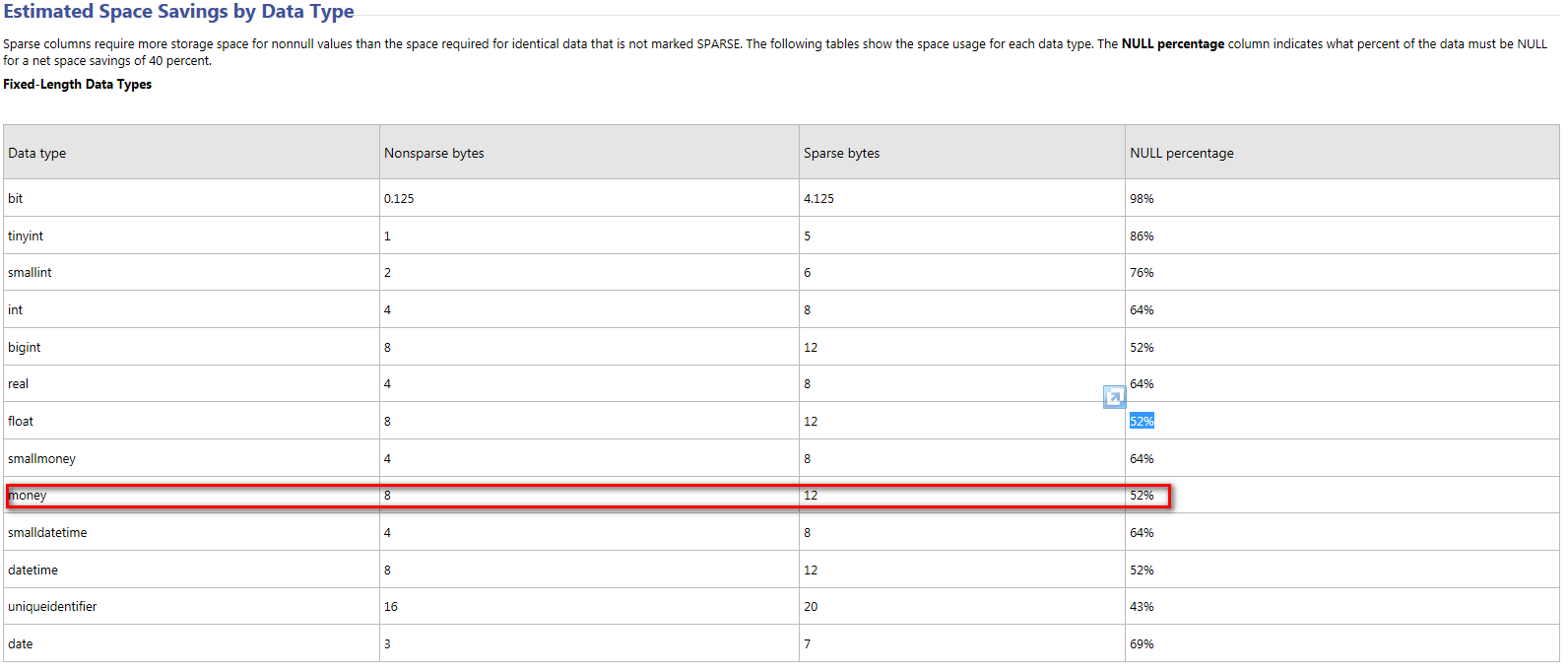This code can be optimized but the different steps above help the comprehension of this functionality.
For my example, this is the changed query :

`ALTER TABLE DimReseller ALTER COLUMN MinPaymentAmount ADD SPARSE`